# 15-344/Homework Assignment 9

This assignment is due at the tutorials on Thursday December 3. Here and everywhere, neatness counts!! You may be brilliant and you may mean just the right things, but if the teaching assistants will be having hard time deciphering your work they will give up and assume it is wrong.

Solve problems 2abde, 2c, 4abc, 4d, 12, 16, 20, and 25 in section 6.1 and problems 1, 2, 11abcd, 11e, 30, 32, and 43 in section 6.2, but submit only your solutions of the underlined problems.

In addition, solve the following problem. There is no need to submit your solution.

Part 1. For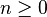$n\geq 0$, let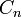$C_n$ be the$n$'th Catalan number, defined as the number of words made of$n$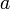$a$'s and$n$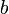$b$'s in which in every initial segment there are at least as many$a$'s as$b$'s. Prove that for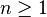$n\geq 1$,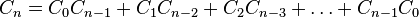$C_n=C_0C_{n-1}+C_1C_{n-2}+C_2C_{n-3}+\ldots+C_{n-1}C_0$.

Hint. If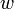$w$ is such a word, let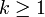$k\geq 1$ be the smallest such that if you cut$w$ after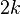$2k$ letters, then the number of$a$'s before the cut is equal to the number of$b$'s before the cut. What can you say about the part of$w$ before the cut, and the part of$w$ after the cut?

Part 2. For$n\geq 1$, let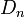$D_n$ be the number of different ways of computing the product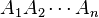$A_1A_2\cdots A_n$ of$n$ matrices. Prove that for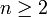$n\geq 2$,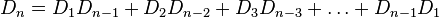$D_n=D_1D_{n-1}+D_2D_{n-2}+D_3D_{n-3}+\ldots+D_{n-1}D_1$.

Hint. In the last matrix multiplication to be carried out, you'd be multiplying the product of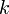$k$ of the matrices with the product of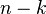$n-k$ of the matrices.

Part 3. Let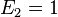$E_2=1$ and for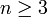$n\geq 3$ let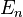$E_n$ be the number of triangulations of a convex$n$-gon using non-crossing diagonals. Prove that for$n\geq 3$,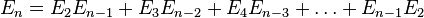$E_n=E_2E_{n-1}+E_3E_{n-2}+E_4E_{n-3}+\ldots+E_{n-1}E_2$.

Hint. Choose one edge of the$n$-gon, and consider the triangle on top of it, what's to its left, and what's to its right.

Part 4. Use the above three assertions to prove that for any$n\geq 0$,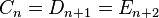$C_n=D_{n+1}=E_{n+2}$.

 Dror's notes above / Students' notes below

Homework Assignment 9 Solution

2) Build a generating function for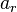$a_{r}$, the number of integer solutions to the following equations.

c)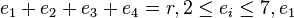$e_{1}+e_{2}+e_{3}+e_{4} = r, 2\leq e_{i} \leq 7, e_{1}$ even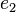$e_{2}$ odd.

A) For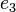$e_{3}$ and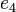$e_{4}$ together, we have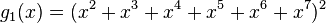$g_{1}(x) = (x^2+x^3+x^4+x^5+x^6+x^7)^2$

For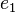$e_{1}$, we have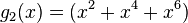$g_{2}(x) = (x^2+x^4+x^6)$

For$e_{2}$, we have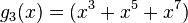$g_{3}(x) = (x^3+x^5+x^7)$

In total, we have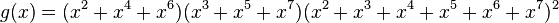$g(x) = (x^2+x^4+x^6)(x^3+x^5+x^7)(x^2+x^3+x^4+x^5+x^6+x^7)^2$

4 d) Three different boxes with at most five objects in the first box

A)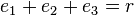$e_{1}+e_{2}+e_{3} = r$ with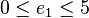$0\leq e_{1} \leq 5$

For$e_{1}$, we have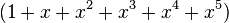$(1+x+x^2+x^3+x^4+x^5)$

Note that for the other two, we don't have a limit, so: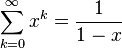$\sum_{k=0}^{\infty}x^{k} = \frac{1}{1-x}$

so, in total we have: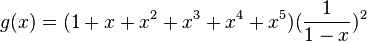$g(x) = (1+x+x^2+x^3+x^4+x^5)(\frac{1}{1-x})^2$

16) Find a generating function for the number of integers between 0 and 999,999 whose sum of digits is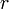$r$.

A) For each integer in the range, we can express them as a six digit number, including 0's in the front (5 will be 000005). So this amounts to finding: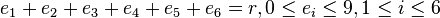$e_{1}+e_{2}+e_{3}+e_{4}+e_{5}+e_{6} = r , 0 \leq e_{i} \leq 9 , 1 \leq i \leq 6$

So, we have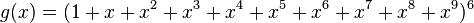$g(x) = (1+x+x^2+x^3+x^4+x^5+x^6+x^7+x^8+x^9)^6$

Section 6.1 2) Find the coefficient of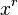$x^r$ in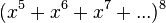$(x^5 + x^6 + x^7 +...)^8$

A)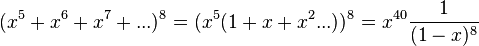$(x^5 + x^6 + x^7 +...)^8 = (x^5(1+ x + x^2 ...))^8 = x^{40} \frac{1}{(1-x)^8}$

The coefficient of$x^r$ in this will be of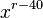$x^{r-40}$ in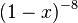$(1-x)^{-8}$, which is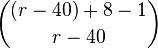$\binom{(r-40) + 8 - 1}{r-40}$